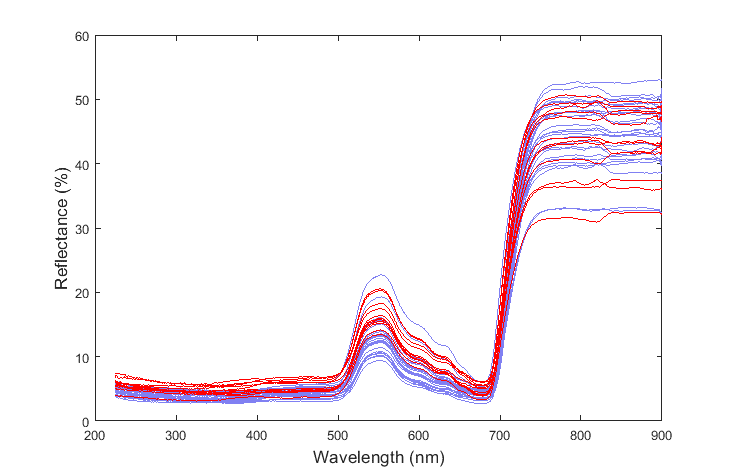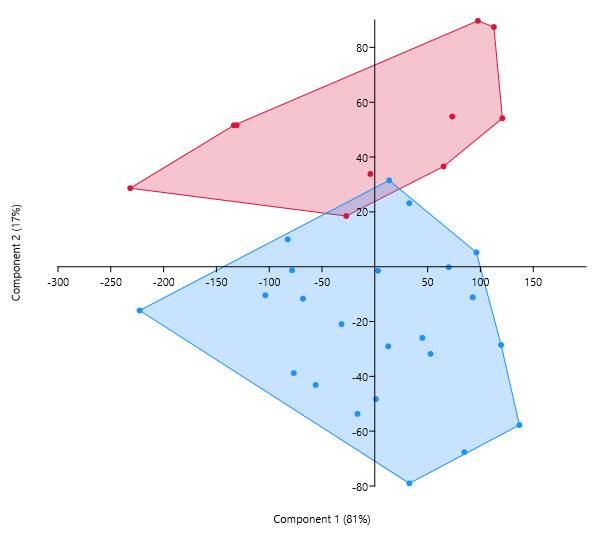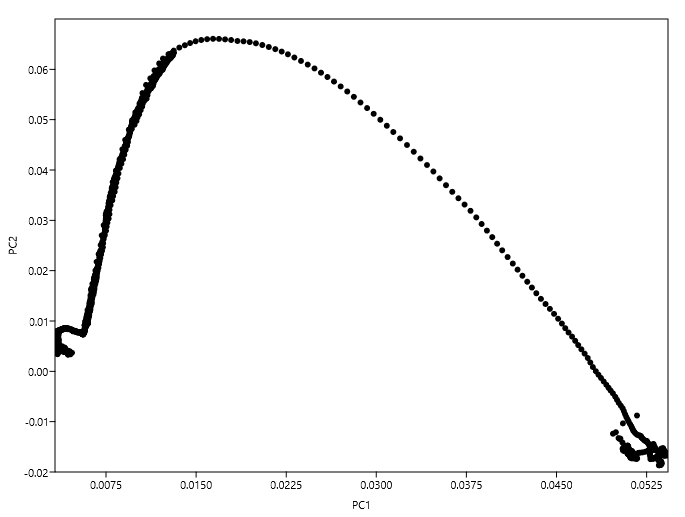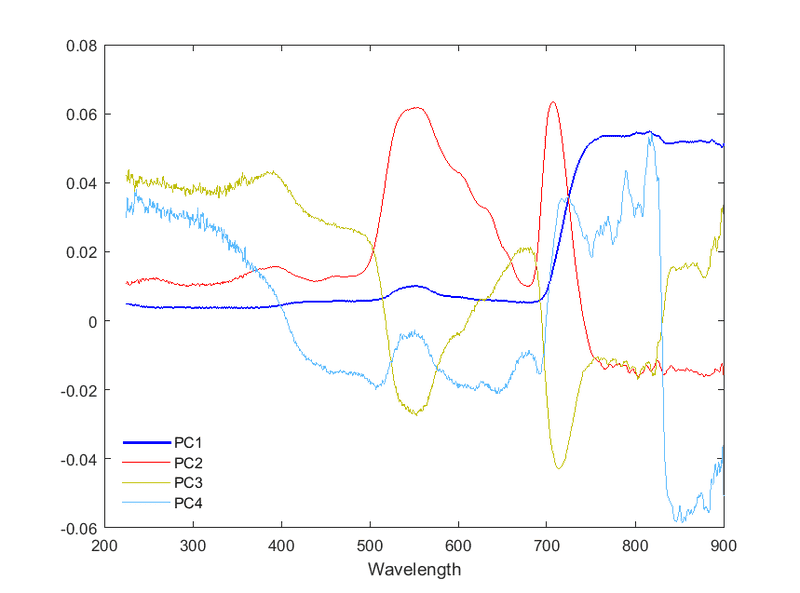# Using PCA for variable reduction

• I
In the textbook “Principal Component Analysis” Jolliffe (§9.2) suggests the following method for variable reduction:

When the variables fall into well-defined clusters, there will be one high-variance PC and, except in the case of 'single-variable' clusters, one or more low-variance PCs associated with each cluster of variables. Thus, PCA will identify the presence of clusters among the variables, and can be thought of as a competitor to standard cluster analysis of variables. [...] Identifying clusters of variables may be of general interest in investigating the structure of a data set but, more specifically, if we wish to reduce the number of variables without sacrificing too much information, then we could retain one variable from each cluster.”

I am trying to apply this idea of variable reduction to a problem which involves differentiating two substances based on their spectra which is composed of 1350 variables (i.e. wavelengths):And here is the resulting differentiation between the species:I want to be able to perform the same differentiation, but using fewer variables. Unfortunately, the textbook does not give any examples of how to do this. The aim is to divide variables, rather than observations, into groups. So, how should we plot the variables in order to see the clusters?For instance, I have tried to plot the loadings at each variable with respect to the first two PCs and obtained:In this plot there doesn't seem to be any well-defined clusters to choose variables from. Am I following Jolliffe's method correctly?

Any explanation would be greatly appreciated.

P.S. Plotting the actual PCs give the following (the ordinate should represent the correlation coefficients between a given variable and the PC):#### Attachments

•Klystron

Stephen Tashi
In the textbook “Principal Component Analysis” Jolliffe (§9.2) suggests the following method for variable reduction:

When the variables fall into well-defined clusters, there will be one high-variance PC and, except in the case of 'single-variable' clusters, one or more low-variance PCs associated with each cluster of variables.

It's unclear to me what the quoted passage means by "the variables". On the one hand, "the variables" might mean the variables in the raw data. On other hand "the variables" might be the coefficients for the principal components that are associated with a leaf.

A principal component is a vector and it is a constant vector. So the terminology "high variance PC" is imprecise. I interpret it to mean that the coefficient associated with that vector (considered as a random variable varying over the population of items in the data) has high variance.

•Klystron
Ygggdrasil
Gold Member
And here is the resulting differentiation between the species:In the graph above, it seems you have already achieved variable reduction. Instead of each spectra being represented by a vector with 1350 observations, the above plot represents each spectra with just two variables (PC1 and PC2). Furthermore, the difference between the red and blue spectra seems to along PC2, with high values of PC2 associated with your red spectra and low values of PC2 associated with the blue spectra.

#### Attachments

It's unclear to me what the quoted passage means by "the variables". On the one hand, "the variables" might mean the variables in the raw data. On other hand "the variables" might be the coefficients for the principal components that are associated with a leaf.

It is referring to the variables in the raw data.

Here is a paper by the same author that also mentions this "cluster criterion" technique for selecting a subset of the original variables in the raw data. But the problem is that they don't give a clear worked example, so I am not sure how to plot the data to see clusters in the variables.

Are they suggesting making a dendrogram based on some kind of cluster analysis of the variables?In the graph above, it seems you have already achieved variable reduction. Instead of each spectra being represented by a vector with 1350 observations, the above plot represents each spectra with just two variables (PC1 and PC2). Furthermore, the difference between the red and blue spectra seems to along PC2, with high values of PC2 associated with your red spectra and low values of PC2 associated with the blue spectra.

Hi Ygggdrasil,

This is true. But I have used all 1350 variables (i.e., wavelengths) to conduct PCA and differentiate the species. And in my application, 1350 variables are too many. So I want to select a subset of these variables/wavelengths to retain. The technique suggested above by Jolliffe is meant to be a criterion for selecting the best subset of variables. Unfortunately, I couldn't find a guide on how to implement this technique.

Last edited:
Ygggdrasil
Gold Member
This is true. But I have used all 1350 variables (i.e., wavelengths) to conduct PCA and differentiate the species. And in my application, 1350 variables are too many. So I want to select a subset of these variables/wavelengths to retain. The technique suggested above by Jolliffe is meant to be a criterion for selecting the best subset of variables. Unfortunately, I couldn't find a guide on how to implement this technique.

In this case, I'd select the wavelengths with the largest loading in PC2 (which would be the wavelengths around 550nm and 700nm). Looking at the spectra, it does seem to be the case that the red spectra tend to have higher reflectance at those wavelengths than the blue spectra.

•roam
In this case, I'd select the wavelengths with the largest loading in PC2 (which would be the wavelengths around 550nm and 700nm). Looking at the spectra, it does seem to be the case that the red spectra tend to have higher reflectance at those wavelengths than the blue spectra.

So, what if the separation is mainly in terms of PC2, but some of the peak regions of that PC curve have negative values? Would you use wavelengths that have negative loadings?For instance, in the above, the PCA was done with the covariance matrix of the variables. If I recompute the PCA using the correlation matrix, the separation is still in terms of PC2, but I get totally different values for the loadings. Some of the peaks of PC2 will have negative values. How should we treat these regions?

Ygggdrasil
So, what if the separation is mainly in terms of PC2, but some of the peak regions of that PC curve have negative values? Would you use wavelengths that have negative loadings?•